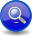#Boost C++ Libraries

...one of the most highly regarded and expertly designed C++ library projects in the world.

##### Character Parser (`char_`, `lit`)
###### Description

The `char_` parser matches single characters. The `char_` parser has an associated Character Encoding Namespace. This is needed when doing basic operations such as inhibiting case sensitivity and dealing with character ranges.

There are various forms of `char_`.

###### char_

The no argument form of `char_` matches any character in the associated Character Encoding Namespace.

```char_               // matches any character
```
###### char_(ch)

The single argument form of `char_` (with a character argument) matches the supplied character.

```char_('x')          // matches 'x'
char_(L'x')         // matches L'x'
char_(x)            // matches x (a char)
```
###### char_(first, last)

`char_` with two arguments, matches a range of characters.

```char_('a','z')      // alphabetic characters
char_(L'0',L'9')    // digits
```

A range of characters is created from a low-high character pair. Such a parser matches a single character that is in the range, including both endpoints. Note, the first character must be before the second, according to the underlying Character Encoding Namespace.

Character mapping is inherently platform dependent. It is not guaranteed in the standard for example that ```'A' < 'Z'```, that is why in Spirit2, we purposely attach a specific Character Encoding Namespace (such as ASCII, ISO-8859-1) to the `char_` parser to eliminate such ambiguities.

NoteSparse bit vectors To accommodate 16/32 and 64 bit characters, the char-set statically switches from a `std::bitset` implementation when the character type is not greater than 8 bits, to a sparse bit/boolean set which uses a sorted vector of disjoint ranges (`range_run`). The set is constructed from ranges such that adjacent or overlapping ranges are coalesced. `range_runs` are very space-economical in situations where there are lots of ranges and a few individual disjoint values. Searching is O(log n) where n is the number of ranges.
###### char_(def)

Lastly, when given a string (a plain C string, a `std::basic_string`, etc.), the string is regarded as a char-set definition string following a syntax that resembles posix style regular expression character sets (except that double quotes delimit the set elements instead of square brackets and there is no special negation ^ character). Examples:

```char_("a-zA-Z")     // alphabetic characters
char_("actgACTG")   // DNA identifiers
char_("\x7f\x7e")   // Hexadecimal 0x7F and 0x7E
```
###### lit(ch)

`lit`, when passed a single character, behaves like the single argument `char_` except that `lit` does not synthesize an attribute. A plain `char` or `wchar_t` is equivalent to a `lit`.

Note`lit` is reused by both the string parsers and the char parsers. In general, a char parser is created when you pass in a character and a string parser is created when you pass in a string. The exception is when you pass a single element literal string, e.g. `lit("x")`. In this case, we optimize this to create a char parser instead of a string parser.

Examples:

```'x'
lit('x')
lit(L'x')
lit(c) // c is a char
```
```// forwards to <boost/spirit/home/qi/char/char.hpp>
#include <boost/spirit/include/qi_char_.hpp>
```

Also, see Include Structure.

###### Namespace

Name

```boost::spirit::lit // alias: boost::spirit::qi::lit```

`ns::char_`

In the table above, `ns` represents a Character Encoding Namespace.

###### Model of

Notation

`c`, `f`, `l`

A literal char, e.g. `'x'`, `L'x'` or anything that can be converted to a `char` or `wchar_t`, or a Lazy Argument that evaluates to anything that can be converted to a `char` or `wchar_t`.

`ns`
`cs`

A String or a Lazy Argument that evaluates to a String that specifies a char-set definition string following a syntax that resembles posix style regular expression character sets (except the square brackets and the negation `^` character).

`cp`

A char parser, a char range parser or a char set parser.

###### Expression Semantics

Semantics of an expression is defined only where it differs from, or is not defined in `PrimitiveParser`.

Expression

Semantics

`c`

Create char parser from a char, `c`.

`lit(c)`

Create a char parser from a char, `c`.

`ns::char_`

Create a char parser that matches any character in the `ns` encoding.

`ns::char_(c)`

Create a char parser with `ns` encoding from a char, `c`.

```ns::char_(f, l)```

Create a char-range parser that matches characters from range (`f` to `l`, inclusive) with `ns` encoding.

`ns::char_(cs)`

Create a char-set parser with `ns` encoding from a char-set definition string, `cs`.

`~cp`

Negate `cp`. The result is a negated char parser that matches any character in the `ns` encoding except the characters matched by `cp`.

###### Attributes

Expression

Attribute

`c`

`unused` or if `c` is a Lazy Argument, the character type returned by invoking it.

`lit(c)`

`unused` or if `c` is a Lazy Argument, the character type returned by invoking it.

`ns::char_`

The character type of the Character Encoding Namespace, `ns`.

`ns::char_(c)`

The character type of the Character Encoding Namespace, `ns`.

```ns::char_(f, l)```

The character type of the Character Encoding Namespace, `ns`.

`ns::char_(cs)`

The character type of the Character Encoding Namespace, `ns`.

`~cp`

The attribute of `cp`.

###### Complexity

O(N), except for char-sets with 16-bit (or more) characters (e.g. `wchar_t`). These have O(log N) complexity, where N is the number of distinct character ranges in the set.

###### Example
NoteThe test harness for the example(s) below is presented in the Basics Examples section.

Some using declarations:

```using boost::spirit::qi::lit;
using boost::spirit::ascii::char_;
```

Basic literals:

```test_parser("x", 'x');                      // plain literal
test_parser("x", lit('x'));                 // explicit literal
test_parser("x", char_('x'));               // ascii::char_
```

Range:

```char ch;
test_parser_attr("5", char_('0','9'), ch);  // ascii::char_ range
std::cout << ch << std::endl;               // prints '5'
```

Character set:

```test_parser_attr("5", char_("0-9"), ch);    // ascii::char_ set
std::cout << ch << std::endl;               // prints '5'
```

Lazy char_ using Phoenix

```namespace phx = boost::phoenix;
test_parser("x", phx::val('x'));            // direct
test_parser("5",
char_(phx::val('0'),phx::val('9')));    // ascii::char_ range
```